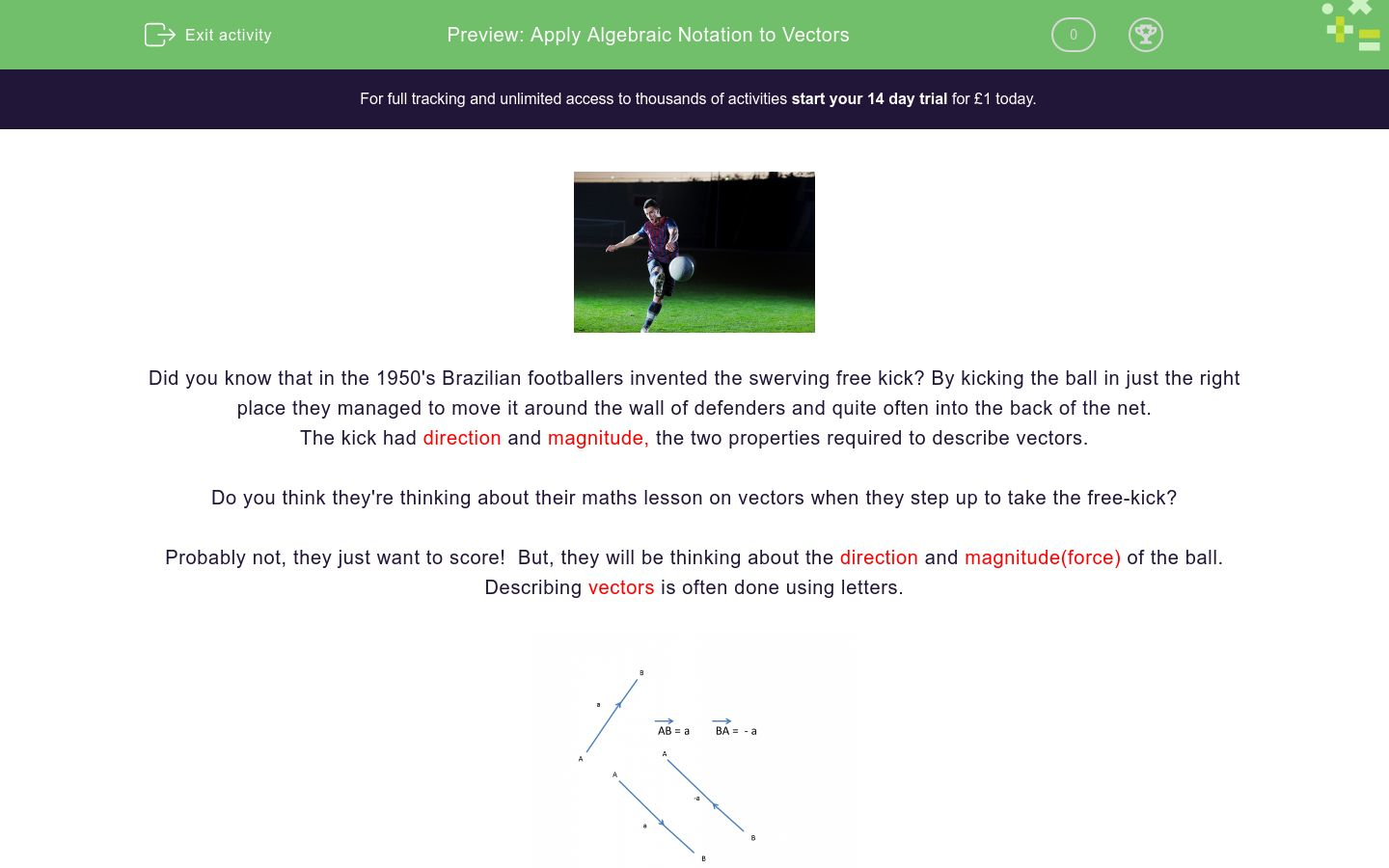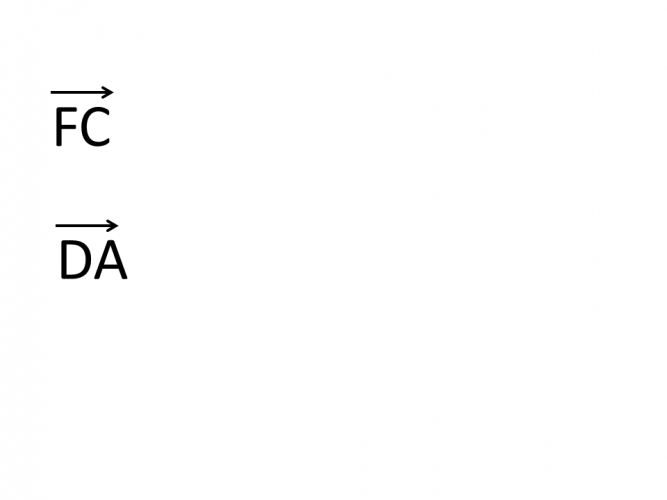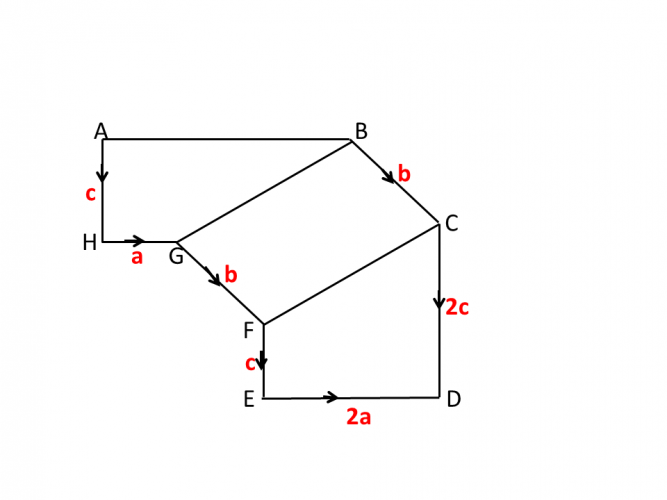# Apply Algebraic Notation to Vectors

In this worksheet, students will learn the notation for vectors using algebra and be able to apply it when describing vectors.Key stage:  KS 4

GCSE Subjects:   Maths

GCSE Boards:   AQA, Eduqas, Pearson Edexcel, OCR

Curriculum topic:   Geometry and Measures, Congruence and Similarity

Curriculum subtopic:   Vectors, Plane Vector Geometry

Difficulty level:### QUESTION 1 of 10Did you know that in the 1950's Brazilian footballers invented the swerving free kick? By kicking the ball in just the right place they managed to move it around the wall of defenders and quite often into the back of the net.

The kick had direction and magnitude, the two properties required to describe vectors.

So when the footballers are shouting things when about to take a free kick, do you think they remember vector work from school?

Probably not, they just want to score the goal.  But they will be thinking about the direction and magnitude(force) of the ball.

Describing vectors is often done using letters.Vectors are written as small case letter and in bold.

Vectors are shown in the direction of the arrow.

In the example above A to B = a but if you went from B to A you would be going in the opposite direction therefore -a.

Lets have a lookIn the diagram above it shows thatTherefore

WY    = 2b   but YW = -2b as you would be moving in the opposite direction to b.

MH    = 2b  + a.  Move along the horizontal first and then the vertical

RL   = -3b + a OR  a -3bLook at the diagram and make some up of your own.

Note:

You can only travel by known vectors.

Lets go and get one over that wall of defenders.→SB = a  and

→ ST = b,

Work out the vector  →CR

-a -2b

-2b-a

2a - b

2b - a

Here is a regular hexagon.→AB = a  and   →BC = b

Which of the following describe the vectors below?-a -2b

-2b-a

2a - b

2b - aCalculate the vector → ECCalculate the vector → FA

 4b - b + a 4a - b - a -2b Vector →FAMatch up.

Start at the letter Q each time, state where you are after following the vectors given.

## Column B

2b - a
F
3b + 2a
S
a - 2b
K
-a + 2b
L
2a - 2b
V
-2b - a
ZCalculate vector → BACalculate the vector  →AB

2a - 2b + c

3a + b

2a + 2b + 2c

3aMZ JZ JS YH vector 3a - 3b =The points P, Q and R lie on a straight line.

The vector →PQ is 2a + b, where a and b are vectors.

Which of the following vectors could be the vector → PR

2a + 2b

4a + 2b

2a - b

-6a - 3bm is the midpoint between → OM

n is the midpoint between  →OC.

What is the vector AN?

• Question 1→SB = a  and

→ ST = b,

Work out the vector  →CR

-a -2b
-2b-a
EDDIE SAYS
Here you could go either of two ways. You can only move horizontally or vertically. Look at the direction of the arrow. Are you going in the same direction? In this example you are not so the vectors are negative.
• Question 2

Here is a regular hexagon.→AB = a  and   →BC = b

Which of the following describe the vectors below?EDDIE SAYS
The word regular hexagon should be a help here. Because the hexagon is regular AD is parallel to BC therefore equal to 2b (DA therefore -2b) and AB is parallel to FC = 2a. Always pay attention to the wording in the questions. There are always clues to help us.
• Question 3Calculate the vector → EC

-b + 4a
EDDIE SAYS
How is your navigation? You have clear guidance here. There is only one way to travel There is nothing from CD or AF to help us. From E to F you are going against the direction shown -b and from F to C you are going in the same direction.
• Question 4Calculate the vector → FA

 4b - b + a 4a - b - a -2b Vector →FA
EDDIE SAYS
Always go via the known route and you can't go wrong. Following instructions is a good thing at times you see!!!
• Question 5Match up.

Start at the letter Q each time, state where you are after following the vectors given.

## Column B

2b - a
S
3b + 2a
F
a - 2b
L
-a + 2b
Z
2a - 2b
K
-2b - a
V
EDDIE SAYS
Its a bit like line dancing. Two steps forward, one step back. See vectors are everywhere. You can only move horizontally or vertically, forward or back.
• Question 6Calculate vector → BA

-2b + a + b
EDDIE SAYS
Did you know you can also simplify vectors? -2b + a + b = b + a. This is the beauty of algebra, we can always make things easier for ourselves which is great if we needed this in a later calculation.
• Question 7Calculate the vector  →AB

3a
EDDIE SAYS
There is a lot to take in here so don\'t try and do this in your head. Write out the vector in full first. c + a + b + c + 2a - 2c - b. Be careful to ensure you are going in the right direction. Then simplify.
• Question 8MZ JZ JS YH vector 3a - 3b =
EDDIE SAYS
Its just a case of counting units and making sure they fit the vector given. You would be given the vector and no starting point...the meanies.
• Question 9The points P, Q and R lie on a straight line.

The vector →PQ is 2a + b, where a and b are vectors.

Which of the following vectors could be the vector → PR

4a + 2b
-6a - 3b
EDDIE SAYS
You are given vector PQ and now that PR is on the straight line. You need to work out what to multiply the given vector by. In this instance PQ has been multiplied by 2 to get PR 4a + 2b. and also multiplied by -3 to get -6a - 3b. Both sides of the vector need to multiplied up.
• Question 10m is the midpoint between → OM

n is the midpoint between  →OC.

What is the vector AN?

-m + 1/2n
EDDIE SAYS
Don\'t be put off by mid points, they are just half the distance. Yes you can use fractions when writing vectors. You will need to do this when it comes to vector proofs.
---- OR ----

Sign up for a £1 trial so you can track and measure your child's progress on this activity.

### What is EdPlace?

We're your National Curriculum aligned online education content provider helping each child succeed in English, maths and science from year 1 to GCSE. With an EdPlace account you’ll be able to track and measure progress, helping each child achieve their best. We build confidence and attainment by personalising each child’s learning at a level that suits them.

Get started